# PySpark 组件

Spark 组件为使用 Python 的 Spark 用户提供服务，用户通过 Python 编写 Spark 应用程序，通过 PySpark 组件完成部署。本文介绍部分使用方法（更多用法请参考 社区指引）。

PySpark 包含标准 Spark 的功能，同时支持上传 Python 脚本、实时修改脚本和 SQL 功能，更加灵活，推荐您使用 PySpark 进行数据预处理。

## 版本说明

PySpark 组件中使用的 Python 版本和支持的第三方模块版本信息如下：

• Python 2.7.5
• SciPy 0.12.1
• NumPy 1.7.1

``````import pip
pip.main(['install', "package_name"])``````

## 操作步骤

1. 添加组件
从左侧菜单栏中，选择【组件】>【机器学习】 列表下的 PySpark 节点，并将其拖拽至画布中。
2. 配置参数
• 脚本及依赖包文件上传：
将任务脚本上传至程序脚本框。如果需要依赖文件，则压缩为 zip 文件后通过依赖包文件框上传。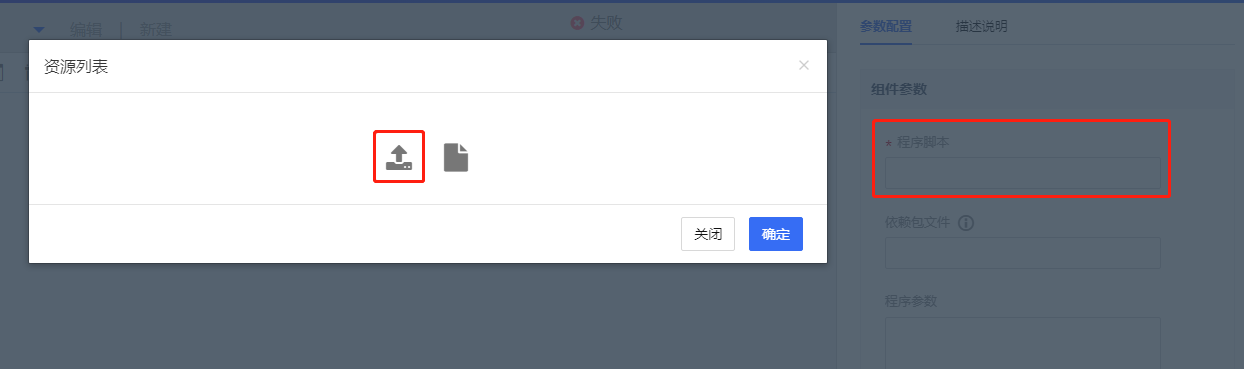• 算法参数：指定您的 PySpark 应用程序所需的参数，即传给 PySpark 脚本的参数，可选项。
• 配置资源：指定您的 PySpark 应用程序用到的配置文件，可选项。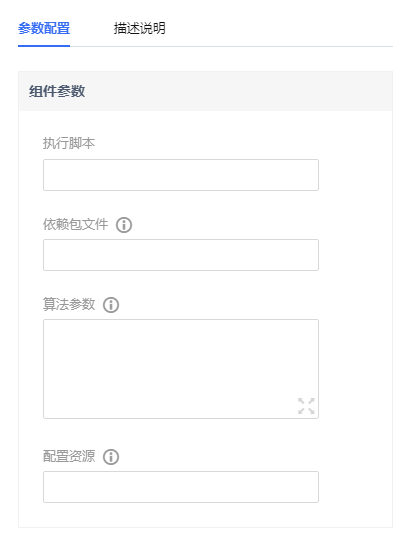3. 配置资源
在【资源参数】列表框配置任务的资源参数。
• num-executors：指定分配的 Executor 个数。
• driver-memory：指定 Driver 需要的内存大小，单位为 GB。
• executor-cores：指定每个 Executor 上需要的 CPU Core 数。
• executor-memory：指定每个 Executor 上需要的内存大小，单位为 GB。
• spark-conf：指定 Spark 的属性参数，换行分割，例如 spark.default.parallelism=200。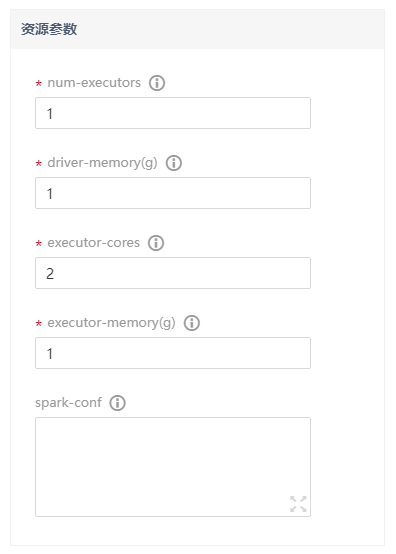4. 运行
单击【保存】并运行工作流。
5. 查看 PySpark 控制台和日志
在 PySpark 节点上单击右键菜单，可查看任务状态和详细日志。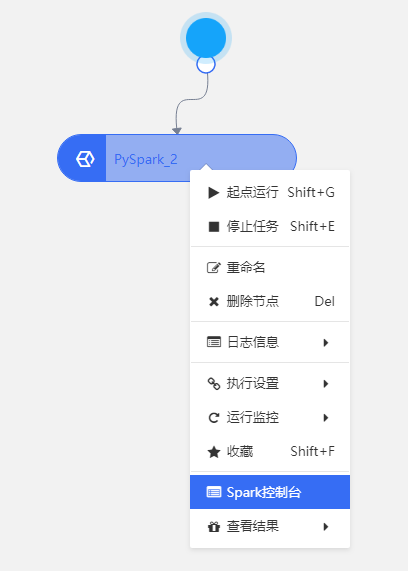详细日志如下：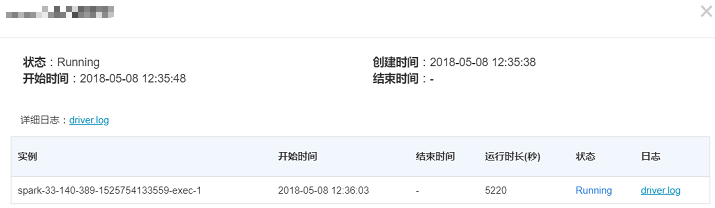## 使用建议

#### 使用 Spark 的 DataFrame，而不要使用 Pandas 的 DataFrame

PySpark 本身就具有类似 pandas.DataFrame 的 DataFrame，所以直接使用 PySpark 的 DataFrame 即可，基于 PySpark 的 DataFrame 的操作都是分布式执行的，而 pandas.DataFrame 是单机执行的，例如：

``````...
df.show()
# +----+-------+
# | age|   name|
# +----+-------+
# |null|Michael|
# |  30|   Andy|
# |  19| Justin|
# +----+-------+

pandas_df = df.toPandas() # 将 PySpark 的 DataFrame 转换成 pandas.DataFrame，并获取'age'列
age = pandas_df['age']
...``````

df.toPandas() 操作会将分布在各节点的数据全部收集到 Driver上，再转成单机的 pandas.DataFrame 数据结构，适用于数据量很小的场景，如果数据量较大时，则此方法不可取。
PySpark的DataFrame 本身支持很多操作，直接基于它实现后续的业务逻辑即可，例如上述代码可以改成`age = df.select('age')`

#### 在 Task 里使用 Python 库，而不是在 Driver上 使用 Python 库

`````` from sklearn import preprocessing
data = np.array(rdd.collect(), dtype=np.float)
normalized = preprocessing.normalize(data)``````

``````from pyspark.ml.feature import Normalizer

# Normalize each Vector using \$L^1\$ norm.
normalizer = Normalizer(inputCol="features", outputCol="normFeatures", p=1.0)
l1NormData = normalizer.transform(dataFrame)``````

``````# record -> other record
def process_fn(record):
# your process logic
# for example
# import numpy as np
# x = np.array(record, type=np.int32)
# ...

# record -> True or Flase
def judge_fn(record):
# return True or Flase

processed = rdd.map(process_fn).map(lambda x: x[1:3])
filtered = processed.filter(judge_fn)``````

process_fn 或 judge_fn 会分发到每个节点上分布式执行，您可以在 process_fn 或 judge_fn 中使用任何 Python 库（如 numpy、scikit-learn 等）。